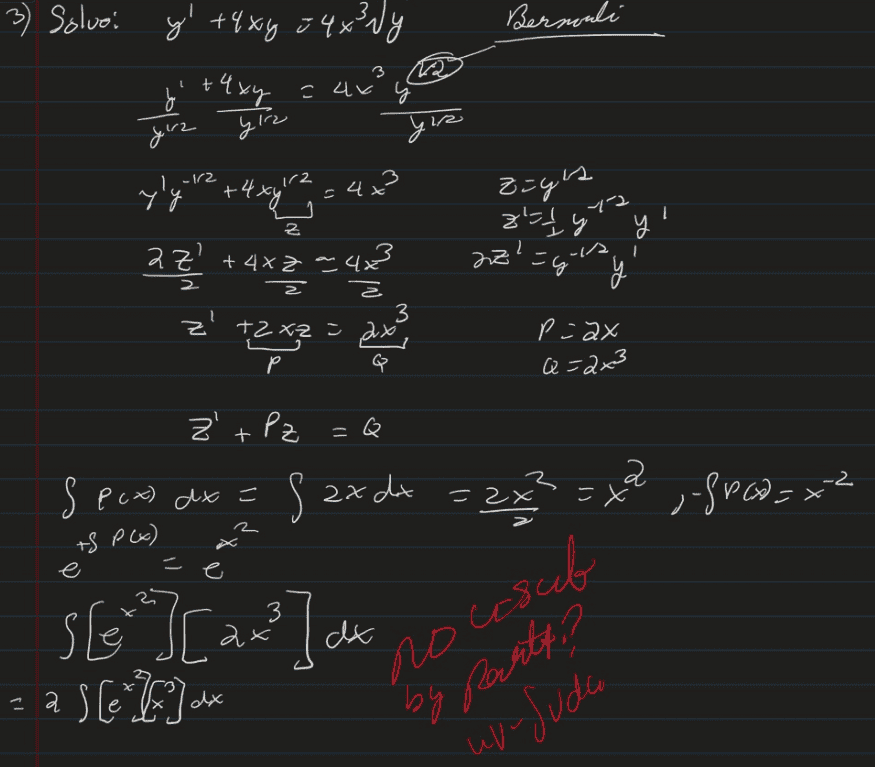# Bernoulli Equation with weird integral

• I
• acalcstudent

#### acalcstudent

TL;DR Summary
Differential equation ending with an integral that doesn't make sensePart of me thinks this is could be a u-sub b/c x^3's derivative is 3x^2, a factor of 3 off from what e is raised to...but it is not a traditional u-sub...any thoughts if this is a u-sub or by parts, and what u should be?

I know that there is more to solving the equation after this ( z = e^{1/(x^2)}(c_1+[insert integral from above], y = z^2) but i can't get to that without the integral above.

Last edited:

$$z'+Pz=Q$$
$$-\int p(x)dx=-x^{2}$$ and not what you wrote down which was $x^{-2}$. you could have cleverly spotted that multiplying throughout by $e^{x^{2}}$ the LHS is a derivative. Integrating the RHS can be written (with the appropriate change of variable (which you can find for yourself)):
$$\frac{1}{2}\int ue^{u}du$$